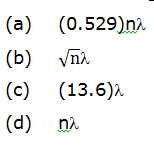# Consider an electron in the nth orbit of a hydrogen atom in the Bohr model. The circumference of the orbit can be expressed in terms of de-Broglie wavelength  of that electron as8 years ago

(d)

The circumference of an orbit in an atom in terms of wavelength of wave associated with electron is given by the relation, circumference = nl

where, n = 1,2,3, ……………………